2 views

I have a huge file in HDFS having Time Series data points (Yahoo Stock prices).

I want to find the moving average of the Time Series how do I go about writing the Apache Spark job to do that .

by (32.3k points)
edited

Moving average is a very tricky task for Spark, and any distributed system.

In our approach we have to duplicate the data at the start of the partitions, so that calculating the moving average per partition gives complete coverage.

Here is a way to do this in Spark. The example data: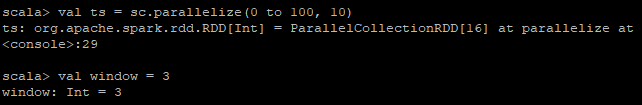A simple partitioner that puts each row in the partition we specify by the key: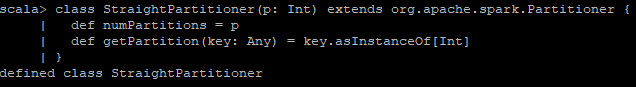Create the data where the first window - 1 rows is copied to the previous partition: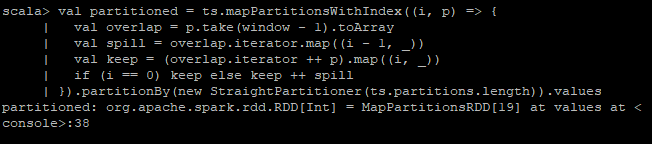Just calculate the moving average on each partition: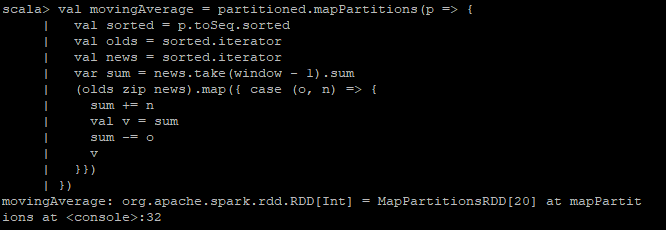Because of the duplicate segments this will have no gaps in coverage.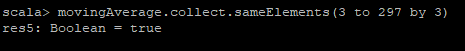If you want to know more about Spark, then do check out this awesome video tutorial: